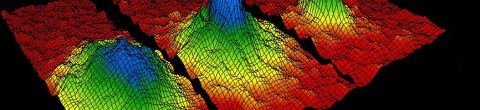# Statistical MechanicsSpring, 2013

Statistical mechanics is a branch of physics that applies probability theory to the study of the thermodynamic behavior of systems composed of a large number of particles. Statistical mechanics provides a framework for relating the microscopic properties of individual atoms and molecules to the macroscopic bulk properties of materials that can be observed in everyday life. Thus it explains thermodynamics as a result of the classical and quantum-mechanical descriptions of statistics and mechanics at the microscopic level. (Source: Wikipedia)

## Lectures in this Course

1. ### Entropy and conservation of information

Professor Susskind introduces statistical mechanics as one of the most universal subjects in modern physics in terms of it's ability to explain and predict natural phenomena.  He begins with a brief introduction to probability theory and then moves... [more]
2. ### Temperature

Professor Susskind presents the physics of temperature. Temperature is not a fundamental quantity, but is derived as the amount of energy required to add an incremental amount of entropy to a system.  As the energy of a system increases, the number... [more]
3. ### Maximizing entropy

After reviewing the laws of thermodynamics, Professor Susskind begins the derivation of how energy states are distributed for a complex system system with many energy states. As the number of particles in a system grows, the distribution of states... [more]
4. ### The Boltzmann distribution

Professor Susskind completes the derivation of the Boltzman distribution of states of a system. This distribution describes a system in equilibrium and with maximum entropy. He derives the formulas for energy, entropy, temperature, and the partition... [more]
5. ### Pressure of an ideal gas and fluctuations

Professor Susskind derives the formula for the pressure of an ideal gas.  He begins by introducing the Helmholtz free energy, and the concept of adiabatic processes.  These concepts lead to the definition of pressure as the change of energy with... [more]
6. ### Weakly interacting gases, heat, and work

Professor Susskind derives the equations for the energy and pressure of a gas of weakly interacting particles, and develops the concepts of heat and work which lead to the first law of thermodynamics.
7. ### Entropy vs. reversibility

Professor Susskind begins the lecture with 2 examples: (1) deriving the speed of sound in an ideal  gas; and (2) a single harmonic oscillator in a heat bath.  The harmonic oscillator example leads to a discrepancy with empirical observation that can... [more]
8. ### Entropy, reversibility, and magnetism

Professor Susskind develops the equation for the probability that all molecules of a gas will converge in one half of a room, and concludes that this event is possible, but that the time scale for it to occur is incredibly long.  This line of... [more]
9. ### Tbe Ising model

After reviewing the discussion of a single magnetic particle (or spin) in a heat bath, Professor Susskind continues with the development of the one-dimensional Ising model.  This model does not exhibit phase transitions.  He then moves on to the... [more]
10. ### Liquid-gas phase transition

Professor Susskind continues the discussion of phase transitions beginning with a review of the Ising model and the mean field approximation, and then presents the temperature and magnetic field parameters of the phase transition of a magnetic... [more]